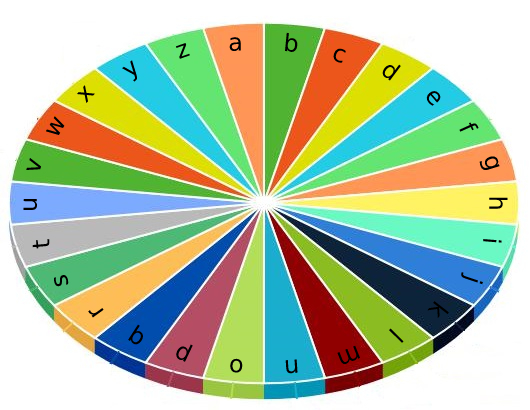# 1974. Minimum Time to Type Word Using Special Typewriter

Easy
There is a special typewriter with lowercase English letters `'a'` to `'z'` arranged in a circle with a pointer. A character can only be typed if the pointer is pointing to that character. The pointer is initially pointing to the character `'a'`.Each second, you may perform one of the following operations:
• Move the pointer one character counterclockwise or clockwise.
• Type the character the pointer is currently on.
Given a string `word`, return the minimum number of seconds to type out the characters in `word`.
Example 1:
Input: word = "abc"
Output:
5
Explanation:
The characters are printed as follows:
- Type the character 'a' in 1 second since the pointer is initially on 'a'.
- Move the pointer clockwise to 'b' in 1 second.
- Type the character 'b' in 1 second.
- Move the pointer clockwise to 'c' in 1 second.
- Type the character 'c' in 1 second.
Example 2:
Input: word = "bza"
Output:
7
Explanation:
The characters are printed as follows:
- Move the pointer clockwise to 'b' in 1 second.
- Type the character 'b' in 1 second.
- Move the pointer counterclockwise to 'z' in 2 seconds.
- Type the character 'z' in 1 second.
- Move the pointer clockwise to 'a' in 1 second.
- Type the character 'a' in 1 second.
Example 3:
Input: word = "zjpc"
Output:
34
Explanation:
The characters are printed as follows:
- Move the pointer counterclockwise to 'z' in 1 second.
- Type the character 'z' in 1 second.
- Move the pointer clockwise to 'j' in 10 seconds.
- Type the character 'j' in 1 second.
- Move the pointer clockwise to 'p' in 6 seconds.
- Type the character 'p' in 1 second.
- Move the pointer counterclockwise to 'c' in 13 seconds.
- Type the character 'c' in 1 second.
Constraints:
• `1 <= word.length <= 100`
• `word` consists of lowercase English letters.

### 解題

Runtime: 0 ms, faster than 100%
Memory Usage: 2 MB, less than 52.17%
func minTimeToType(word string) int {
str := []byte("a" + word)
ans := 0
for i:=1; i<len(str); i++ {
if str[i-1] > str[i] {
ans += min(int(str[i-1] - str[i]), 26 - int(str[i-1] - str[i]))
} else {
ans += min(int(str[i] - str[i-1]), 26 - int(str[i] - str[i-1]))
}
}
return ans + len(word)
}
func min(a, b int) int {
if a < b { return a }
return b
}